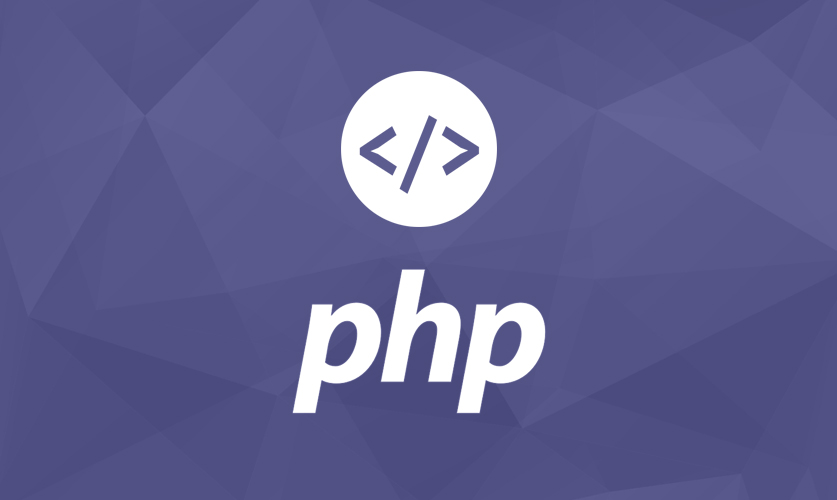# PHP : Function

May 26, 2020A key benefit of using functions is that they are reusable; if you have a task that needs to be performed a number of times, a function is an ideal solution. They can be either defined by you or by PHP (PHP has a rich collection of built-in functions). This article will focus on programmer-defined functions but will touch briefly on PHP’s functions to complete the picture.

```<?php

\$variable1 = 0;

function function1(){
//\$variable1 = 10;
global \$variable1;
return \$variable1;
}

function function2(){
//\$variable1 = 10;
global \$variable1;
return \$variable1;
}

\$result = \$num1 + \$num2;
return \$result;
}

echo function1();
echo function2();

/*PHP The static Keyword*/

function myTest() {
static \$x = 0;
echo \$x;
\$x++;
}

myTest();
myTest();
myTest();

?>```